# SSC CGL EXAMS 2018 | Quantitative Aptitude Practice Questions (Day-17)

Dear Aspirants, Here we have given the Important SSC Exam 2018 Practice Test Papers. Candidates those who are preparing for SSC 2018 can practice these questions to get more confidence to Crack SSC 2018 Examination.

[WpProQuiz 4698]

1) The number of real roots of the equation x2 – 5|x|+6 = 0 is

a) 1

2) 2

c) 3

d) 4

2) 16 sin5 x = P sin 5x + q sin 3x + r sin x. What is the value of (P + r) r + q

a) 511

b) 115

c) (121)5

d) None of these

3) From the top of a lighthouse 153 m high with its base at sea level, the angle of depression of a boat is 150. The distance of the boat from the foot of the light house is?

a) 153 (2 + √3)

b) 76.5 (1 +√3/2 )

c) 153 (2 – √3)

d) 76.5 (1 – √3/2)

4) What is the radius of the circle touching x – axis at (7.25, 0) and y – axis at (0, 7.25)?

a) 14.5 Units

b) 7.25 Units

c) .0 Units

d) 3.62.5 Units

5) The mean of 20 observations is 20. On checking, it was founds that two observations were wrongly copied as 6 and 12. If wrong observations are placed by correct values 18 and 4. Then the correct mean is

a) 20.2

b) 20

c) 21

d) 22.5

6) A water tank has X inlet pipes and y outlet pipes. An inlet pipe can fill the tank is 10 h while on outlet pipe can empty the full tank is 15 h. If all pipes are left open simultaneously it takes 18 h to fill the empty tank. What is the relationship between x and y?

a) x : y = 1 : 4

b) x : y = 4 : 1

c) x : y = 5 : 4

d) x : y = 4 : 5

7) What is the maximum possible value of (31 sin x + 12cos x)?

a) + 15√5

b) 31

c) – 15√5

d) √43

8) PQRS is a quadrilateral. The diagonals of ABCD intersect at the point A. The area of the triangles PAS and QAR are 9 and 36, respectively. If the areas of the triangles PAQ and RAS are equal, then the area of triangle PAS is

a) 18

b) 12

c) 16

d) 10

9) 1319 + 1119 will be divisible by which of the following number?

a) 24

b) 2

c) 3

d) All

10) Find the number of degrees subtended at the center of a circle by an arc, whose length is 19.62 times the radius.

a) 1123.69

b) 1113.5

c) 1125

d) 1024

We have, x2 – 3| x | – 2 |x| + 6 = 0

|x|2 – 3 | x | – 2 | x| + 6 = 0

(|x| – 3) (|x| – 2) = 0

|x| = 3 & | x | = 2

x =± 3 & x = ± 2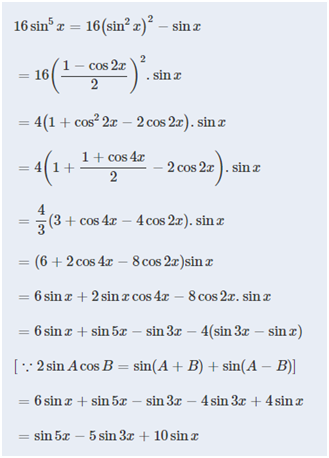By comparison, P = 1, q = – 5, r = 10

(P + r)r + q = (1 + 10)10 – 5

= (11)5

Let the distance of the boat from the foot of lighthouse is x meter.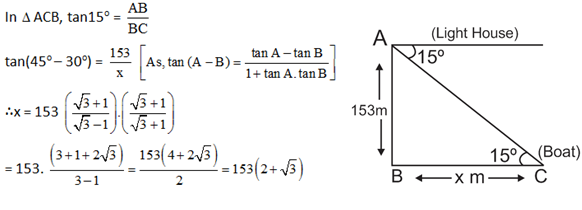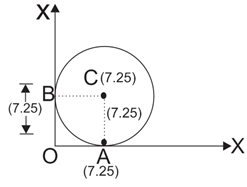Radius of the circle = AC = BC

[As, AC = OB = BC = OA =7.25]

Given that, mean of 20 observations = 20.

Sum of 20 observations = 20*20 = 400

Sum of actual (correct) observations

= 400 – (+ 6 + 12) + (18 + 4) = 400 – 18 + 22 = 404.

Correct mean = 404 / 20 = 20 .2

One hour work of 1 intlet pipe = 1/10

One hour work of x inlet pipes = x/10

One hour work of 1 outlet pipe = 1/15

One hour work of y outlet pipes = y/15

x/10 – y/15 = x – y / 18

(9x – 6y)/90 = 5 (x – y)/ 90

9x – 6y = 5x – 5y

9x – 5x = – 5y + 6y

4x = 1y

x : y = 1 : 4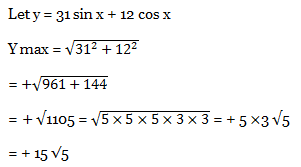We know ratio of area divided by diagonals is same.

9/x = x/36

x2 = 9 * 36

x = 3 * 6 = 18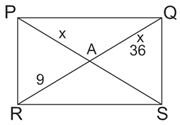xn + yn is divisible by (x + y) where n ϵ odd numbers

So, 1319 + 1119 (13 + 1a) divides it.

24 and its factors also divide it so, 24, 2, 3 all divides it.

Number of radians in the angle,

= 19.620 = 16.62 * 180/π

= 1123.69

### *******************

#### SSC CGL EXAMS 2019 |Quantitative Aptitude Practice Questions (Day-14)